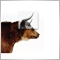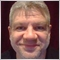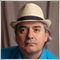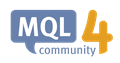# Profit and Loss calculation.1381

Hello,

‌‌ Please any one can help with this:

-I want to know what is the reference symbol closed price used on the MT4 profit and loss calculation.

(‌I always find some differences between my calculations and the MT4 calculations when it comes to the indirect currencies closed trades.)

‌-Where can i find the MT4 API documentation?

R‌egards,41920

Show your calculation.

There is no MT4 API, just mql4.‌1381

Alain Verleyen:

Show your calculation.

There is no MT4 API, just mql4.‌

thank you for the reply.

t‌his is the formula:

A‌mount*[100`000*(close-open)*1/Ref Close)]

f‌or example AUDCAD:   buy 1.0 lot AUDCAD at 2.00000 and close at 2.00001 ,       profit = 1.00 /USDCAD41920

Wasim Zayed:

thank you for the reply.

t‌his is the formula:

A‌mount*[100`000*(close-open)*1/Ref Close)]

f‌or example AUDCAD:   buy 1.0 lot AUDCAD at 2.00000 and close at 2.00001 ,       profit = 1.00 /USDCAD

Seems right, what is the problem ?1381

Alain Verleyen:
Seems right, what is the problem ?

I always get a different result (small fracture) when i close the order on the indirect crosses symbols, when the order is open the floating profit and loss is always the same, but when i close the order i get a different result than the one i have on the MT4 system, so my difference should be connected to the profit and loss formula (i think so). so if possible to get the formula directly from the Meta support so i can use the same formula in my calculations also41920

Wasim Zayed:

I always get a different result (small fracture) when i close the order on the indirect crosses symbols, when the order is open the floating profit and loss is always the same, but when i close the order i get a different result than the one i have on the MT4 system, so my difference should be connected to the profit and loss formula (i think so). so if possible to get the formula directly from the Meta support so i can use the same formula in my calculations also

Please. SHOW your problem with details.1381

Alain Verleyen:
Please. SHOW your problem with details.

I`m using this formula for profit and loss calculation:

Amount*[100000*(Close-Open)*(1/Ref Close)]

According to my calculation using this formula is  -16.41

According to the MT4 calculation the profit is -16.58

I think I misusing the reference number for closing !

Please check the attached screenshot , the numbers are highlighted in RED.

R‌egards,

Files:6581

Wasim Zayed: I`m using this formula for profit and loss calculation:

Amount*[100000*(Close-Open)*(1/Ref Close)]

According to my calculation using this formula is  -16.41

According to the MT4 calculation the profit is -16.58

I think I misusing the reference number for closing !

Please check the attached screenshot , the numbers are highlighted in RED.

Show your true code for the calculations, not just your assumption of what the formula should be, because there might be bugs in your code or misinterpretations of the formula.

Also, you have to consider using either the Bid or Ask price for the conversion depending on whether it is a Buy or a Sell (in other words, consider the spread of the conversion).

By the way, if all you want to do is calculate the Profit in terms of the Deposit/Balance currency, you can just use the OrderProfit() function.‌OrderProfit - Trade Functions - MQL4 Reference
• docs.mql4.com
OrderProfit - Trade Functions - MQL4 Reference41920

Wasim Zayed:

I`m using this formula for profit and loss calculation:

Amount*[100000*(Close-Open)*(1/Ref Close)]

According to my calculation using this formula is  -16.41

According to the MT4 calculation the profit is -16.58

I think I misusing the reference number for closing !

Please check the attached screenshot , the numbers are highlighted in RED.

R‌egards,

1 lot at (1.01412-1.01434) = -22 CAD

Now you need to convert this to USD but you don't say what was the USDCAD rate at this time. (We can see a lot of things on your screenshot but not USDCAD quotation !).‌1381

Alain Verleyen:

1 lot at (1.01412-1.01434) = -22 CAD

Now you need to convert this to USD but you don't say what was the USDCAD rate at this time. (We can see a lot of things on your screenshot but not USDCAD quotation !).‌

In our side we are using the following numbers:

----------------

contract size 100000

open 1.01434

close 1.01412

ref close 1.34029

PL -16.41

F‌ormula

100000 * (1.01412 - 1.01434) *1/1.34029 =

- 16.41435

IN MT SYSTEM we need to know what was the ref close for ticket 356650 to calculate the PL

-----------------

contract size 100000

open 1.01434

close 1.01412

ref close ????

PL ????41920

Wasim Zayed:

IN MT SYSTEM we need to know what was the ref close for ticket 356650 to calculate the PL

-----------------

contract size 100000

open 1.01434

close 1.01412

ref close ????

PL ????

So what ? USDCAD rate is given in Market Watch, you can access it by code. I don't see what is your problem.